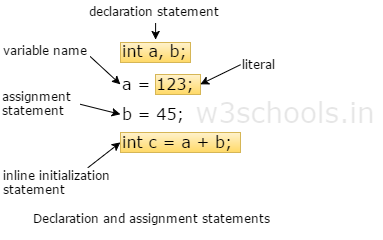Java Variables

Variables are the identifier of the memory location, which used to save data temporarily for later use in the program. During execution of a program, values can be stored in a variable, and the stored value can be changed. In Java programming, it is necessary to declare the variable before being used.

Declaration of Variables in Java

Declaring a variable means what kind of data it will store. Variables display named storage locations, whose values can be changed during the execution of the program. It is the basic unit of storage in a Java program.

Syntax:
type variable_name;
type variable_name, variable_name, variable_name;

Here's the meaning of type is a data type. It specifies what type of data the variable will hold.

Example:
// variable definition
int    width, height=5;
char   letter='C';
float  age, area;
double d;

Initialization of Variables in Java

This means assigning a value to variables. In Java, you can assign a value to variables in two ways:

1. Static - This means that the memory is determined for variables when the program starts.
2. Dynamic - Dynamic means that in Java, you can declare variables anywhere in the program, because when the statement is executed the memory is assigned to them.
Example:
// actual initialization
width = 10;
age = 26.5;The variable name needs to be chosen by the programmer in a meaningful way so that it reflects what it is representing a program.

Rules of Declaring variables in Java

• A variable name can consist of Capital letters A-Z, lowercase letters a-z, digits 0-9, and two special characters such as underscore and dollar Sign.
• The first character must be a letter.
• Blank spaces cannot be used in variable names.
• Java keywords cannot be used as variable names.
• Variable names are case-sensitive.

Scope of Variables in Java

Variable Scope means - That limit, as far as the variable can be used.

In Java there are various types of variable scope:
• Local variables
• Instance variables
• Class/Static variables

Local variables

A variable that is declared within the method that is called local variables. It is defined in method or other statements, such as defined and used within the cache block, and outside the block or method, the variable cannot be used.

Instance variables

A non-static variable that is declared within the class but not in the method is called instance variable. Instance variables are related to a specific object; they can access class variables.

Class/Static variables

A variable that is declared with static keyword in a class but not in the method is called static or class variable.

Example:
class A {
int amount = 100; //instance variable
static int pin = 2315; //static variable

public static void main(String[] args) {
int age = 35; //local variable
}
}
Example:
public class Example {
int salary; // Instance Variable

public void show() {
int value = 2; // Local variable
value = value + 10;
System.out.println("The Value is : " + value);
salary = 10000;
System.out.println("The salary is : " + salary);
}

public static void main(String args[]) {
Example eg = new Example();
eg.show();
}
}